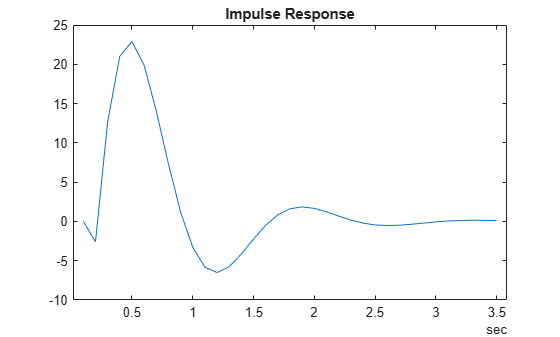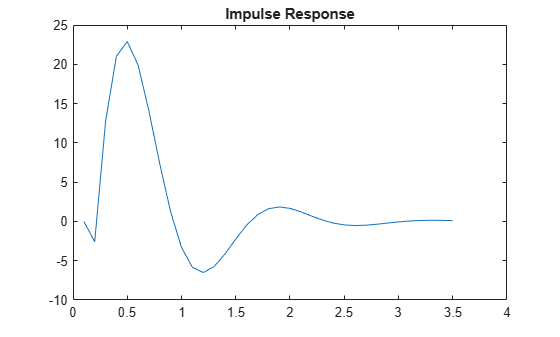# era

Estimate state-space model from impulse response data using Eigensystem Realization Algorithm (ERA)

Since R2022b

## Syntax

``sys = era(data)``
``sys = era(data,nx)``
``sys = era(data,nx,Name=Value)``

## Description

`era` uses the Eigensystem Realization Algorithm  to estimate a state-space model using impulse response data rather than input/output data. `era` is especially useful for identifying dynamic systems for applications such as modal analysis or structural health modeling.You can also use `era` for modeling time-series data for applications such as prediction. For more information about the algorithm, see .

example

````sys = era(data)` estimates a state-space model using the time-domain impulse response data in `data`, which can be either a timetable or matrix that contains only output data. The software determines the order of the model `nx` automatically.`sys` is a model of the following form: $\begin{array}{l}\stackrel{˙}{x}\left(t\right)=Ax\left(t\right)+Bu\left(t\right)+Ke\left(t\right)\\ y\left(t\right)=Cx\left(t\right)+Du\left(t\right)+e\left(t\right)\end{array}$A, B, C, D, and K are state-space matrices. u(t) is the input, y(t) is the output, e(t) is the disturbance, and x(t) is the vector of `nx` states.All entries of A, B, C, and K are free estimable parameters by default. D is fixed to zero by default, meaning that there is no feedthrough, except for static systems (`nx = 0`).The software sets the sample time of `sys` to the sample time of `data` if `data` is a timetable, or to -1 if `data` is a matrix.```

example

````sys = era(data,nx)` specifies the number of states `nx`.```

example

````sys = era(data,nx,Name=Value)` incorporates additional options specified by one or more name-value arguments. For example, use the `Feedthrough` name-value argument to introduce feedthrough by estimating the D matrix. Use the `InputDelay` name-value argument to specify input delays for each channel.```

## Examples

collapse all

Load and plot the data `Htt`, which is a `timetable` that contains impulse response data in the variable `H`.

```load impulseresponse.mat Htt plot(Htt.Time,Htt.H) title('Impulse Response')```Use `era` to estimate a state-space model.

```sys = era(Htt); sysorder = order(sys)```
```sysorder = 2 ```

`sys` is an `idss` model of order 2.

Load and plot the data `H`, which is a numeric matrix. `Ts` is the sample time for the data in `H`.

```load impulseresponse.mat H Ts L = size(H,1); t = (1:L)'*Ts; plot(t,H) title('Impulse Response')```Use `era` to estimate a state-space model of order 3.

```sys = era(H,3); sysorder = order(sys)```
```sysorder = 3 ```

`sys` is an `idss` model of order 3.

Load the impulse response data, which is in the form of a timetable.

`load impulseresponse.mat Htt`

Use `era` to specify a state-space model that includes feedthrough.

`sys = era(Htt,'best',Feedthrough=1);`

## Input Arguments

collapse all

Impulse response data, specified as either a `timetable` with Ns rows and Ny variables or an Ns-by-Ny-by-Nu numeric matrix, where Ns is the number of samples, Ny is the number of outputs, and Nu is the number of inputs. `data` must be uniformly sampled.

• For SISO and SIMO systems, each column i of the timetable or matrix in `data` represents the impulse response from the single input to the ith output.

• For MIMO systems, `data` must be a 3-D matrix. Each Ns-length vector (i,j) of `data` represents the impulse response between output i and input j, `data(:,i,j)`.

`data` must be obtained from a system with zero initial conditions.

Order of the estimated model, specified as a nonnegative integer, a vector containing a range of positive integers, or `"best"`.

• If you already know what order you want your estimated model to have, specify `nx` as a scalar.

• If you want to compare a range of potential orders to choose the most effective order for your estimated model, specify the range in `nx`. `era` creates a Hankel singular-value plot that shows the relative energy contributions of each state in the system. States with relatively small Hankel singular values contribute little to the accuracy of the model and can be discarded with little impact. The index of the highest state you retain is the model order. The plot window includes a suggestion for the order to use. You can accept this suggestion or enter a different order. For an example of using Hankel plots, see `ssest`.

If you do not specify `nx` or if you specify `nx` as `"best"`, the software automatically chooses `nx` from the range `1:10`.

• If you are identifying a static system, set `nx` to `0`.

### Name-Value Arguments

Specify optional pairs of arguments as `Name1=Value1,...,NameN=ValueN`, where `Name` is the argument name and `Value` is the corresponding value. Name-value arguments must appear after other arguments, but the order of the pairs does not matter.

Example: `sys = era(data,2,InputDelay=1)`

Input delay for each input channel, specified as a numeric vector of length equal to the number of inputs in `sys`. To apply the same delay to all channels, specify `InputDelay` as a numeric scalar.

Option to include direct feedthrough from input to output, specified as a logical vector of length Nu, where Nu is the number of inputs. If you specify `Feedthrough` as a logical scalar, that value is applied to all the inputs. The default is `0`, except for static systems, where the software always assumes `Feedthrough` is `1`.

## Output Arguments

collapse all

Identified state-space model, returned as an `idss` array for SISO systems or, for systems with more than one input or output, an array of `idss` models. Each model represents the response of one input to one output. This model is created using the specified model order and delays.

Information about the estimation results and options used is stored in the `Report` property of the model. `Report` has the same fields as an `idss` model that was generated using estimation commands such as `ssest`. However, models generated with `era` do not use all these fields.

Report FieldDescription
`Status`

For `era`-based models, ```Estimated using the Eigensystem Realization Algorithm```

`Method`

`ERA`

`Fit`Quantitative assessment of the estimation, returned as a structure
`Parameters`

Estimated values of model parameters.

`OptionsUsed`

`[]`

`RandState``[]`
`DataUsed`Attributes of the data used for estimation
Termination`[]`

For more information on using `Report`, see Estimation Report.

 Juang, Jer-Nan, and Richard S. Pappa. “An Eigensystem Realization Algorithm for Modal Parameter Identification and Model Reduction.” Journal of Guidance, Control, and Dynamics 8, no. 5 (September 1985): 620–27. https://doi.org/10.2514/3.20031.